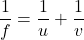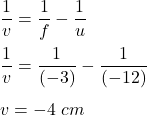Question

a candle is located 12 cm from a concave mirror. The focal point of the mirror is 3.0 cm from the mirror, where is the image located?

1.v = -4 cm

Explanation:

We have,

Distance between candle and the concave mirror, u = -12 cm

Focal length of the mirror is, f = -3 cm

It is required to find the distance from the distance where image is located. Using mirror’s formula to find it:v is image distanceSo, the image will formed at a distance of 4 cm.